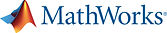Course Highlights

This three-day course provides a comprehensive introduction to the
MATLAB® technical computing environment. No prior programming experience or
knowledge of MATLAB is assumed. Themes of data analysis, visualization, modeling,
and programming are explored throughout the course.

Topics include:

• Working with the MATLAB user interface

• Entering commands and creating variables

• Analyzing vectors and matrices

• Visualizing vector and matrix data

• Working with data files

• Working with data types

• Automating commands with scripts

• Writing programs with branching and loops

• Writing functions

• Automating commands with scripts

Who Should Attend

Engineers, researchers, scientists, and managers who are new to MATLAB or want to update their knowledge on new version of MATLAB.

PartnersTechsource Systems is Mathworks Sole and Authorised Distributor and Training PartnerNil

Course Benefits

Upon the completion of the course, the participants will be able to

- navigate the MATLAB user interface

- create, access and modify vectors and matrices

- perform calculations with vector and matrix data

- visualize vector and matrix data

- import and export data

- write and debug scripts and functions

Working with the MATLAB User Interface

Objective: Become familiar with the main features of the MATLAB integrated design environment and its user interfaces. Get an overview of course themes.

• Plotting data

• Customizing plots

• Exporting graphics for use in other applications

Variables and Commands

Objective: Enter MATLAB commands, with an emphasis on creating variables, accessing and manipulating data in variables, and creating basic visualizations. Collect MATLAB commands into scripts for ease of reproduction and experimentation.

• Entering commands

• Creating numeric and character variables

• Making and annotating plots

• Getting help

• Creating and running live scripts

Analysis and Visualization with Vectors

Objective: Perform mathematical and statistical calculations with vectors. Use MATLAB syntax to perform calculations on whole data sets with a single command. Organize scripts into logical sections for development, maintenance, and publishing.

• Performing calculations with vectors

• Accessing and modifying values in vectors

• Formatting and sharing live scripts

Analysis and Visualization with Matrices

Objective: Use matrices as mathematical objects or as collections of (vector) data. Understand the appropriate use of MATLAB syntax to distinguish between these applications.

• Creating and manipulating matrices

• Performing calculations with matrices

• Calculating statistics with matrix data

• Visualizing matrix data

Tables of Data

Objective: Import data as a MATLAB table. Work with data stored as a table.

• Storing data as a table

• Operating on tables

• Extracting data from tables

• Modifying tables

Conditional Data Selection

Objective: Extract and analyze subsets of data that satisfy given criteria.

• Logical operations and variables

• Finding and counting

• Logical indexing

Organizing Data

Objective: Organize table data for analysis. Represent data using appropriate native MATLAB data types.

• Combining tables of data

• Dates and durations

• Discrete categories

Analyzing Data

Objective: Perform typical data analysis tasks in MATLAB, including importing data from files, preprocessing data, fitting a model to data, and creating a customized visualization of the model.

• Importing from spreadsheets and delimited text files

• Dealing with missing data

• Plotting functions

• Customizing plots

Increasing Automation with Programming Constructs

Objective: Create flexible code that can interact with the user, make decisions, and adapt to different situations.

• Programming constructs

• User interaction

• Decision branching

• Loops

Increasing Automation with Functions

Objective: Increase automation by encapsulating modular tasks as user-defined
functions. Understand how MATLAB resolves references to files and variables. Use
MATLAB development tools to find and correct problems with code

• Creating functions

• Calling functions

• Setting the MATLAB path

• Debugging

• Using breakpoints

• Creating and using structures